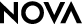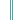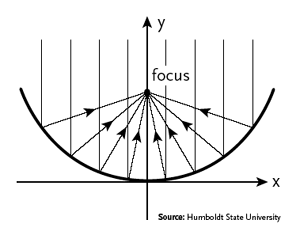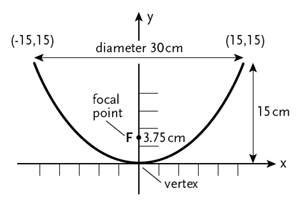Saved By the SunStudent Handout

Finding the Focal Point

If you decide to build a parabolic cooker, you will need to find the focal point of your cooker in order to place your water container where the sun's rays will be strongest.

See It Online
To see how this point changes with the depth of your dish, you can use the Focus of Parabola demonstration from Humboldt State University, in which you can increase or decrease the curvature of a parabola to see how the focal point changes. Find the demonstration at

www.ies.co.jp/math/java/conics/focus/focus.html

Determine It Yourself
You can use the following equation to determine the focal point for yourself.

The formula for a parabola is f = x2/4a. To find the focal point of a parabola, follow these steps:

Step 1: Measure the longest diameter (width) of the parabola at its rim.

Step 2: Divide the diameter by two to determine the radius (x) and square the result (x2).

Step 3: Measure the depth of the parabola (a) at its vertex and multiply it by 4 (4a).

Step 4: Divide the answer from Step 2 by the answer to Step 3 (x2/4a). The answer is the distance from the vertex of the parabola to its focal point.The focal point is the point at which light waves traveling parallel to the axis of the parabola meet after reflecting off its surface.For the above example, the width of a parabola is 30 centimeters, and its depth is 15 centimeters. How far is the focal point from the vertex of the parabola?

x2 = 225 cm2

4a = 60 cm

225 cm2/60 cm = 3.75 cm (focal point)

Note: Each increment on the graph equals 2.5 centimeters.

-->